# Solution assignment 09 Tangent line to graph

### Assignment 9

The graph of the function: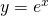intersects the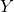-axis in point A.
The tangent line at this point of the graph intersects the-axis. Calculate the coordinates of this intersection point.

### Solution

Intersection point A has the coordinates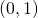. In order to calculate the tangent line at this point, we calculate the slope and we do this by differentiation: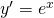and thus the slope in the pointequals: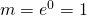The tangent lne has the following equation: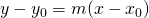with:and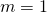and thus: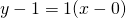or: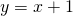This line intersects the-axis in the point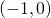.